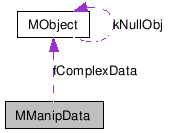# MManipData Class Reference [OpenMayaUI - API module for user interface]

`#include <MManipData.h>`

Collaboration diagram for MManipData:[legend]

## Detailed Description

Manipulator Data.

This class encapulates manipulator data which is returned from the manipulator conversion functions. MManipData is used to represent data that is either simple or complex. Simple data is used to represent bool, short, int, unsigned int, float, and double types. Complex data is used to represent MObjects created by MFnData, or classes derived from MFnData.

Examples:

## Public Member Functions

MManipData ()
~MManipData ()
MManipData (const MManipData &)
MManipData (bool)
MManipData (short)
MManipData (int)
MManipData (unsigned int)
MManipData (float)
MManipData (double)
MManipData (const MObject &)
MManipDataoperator= (const MManipData &)
MManipDataoperator= (bool)
MManipDataoperator= (short)
MManipDataoperator= (int)
MManipDataoperator= (unsigned int)
MManipDataoperator= (float)
MManipDataoperator= (double)
MManipDataoperator= (const MObject &)
bool isSimple () const
bool asBool () const
short asShort () const
int asLong () const
unsigned int asUnsigned () const
float asFloat () const
double asDouble () const
MObject asMObject () const

## Constructor & Destructor Documentation

 MManipData::MManipData ( )

The default class constructor.

 MManipData::~MManipData ( )

The destructor.

 MManipData::MManipData ( const MManipData & other )

The copy constructor.

Parameters:
 [in] other Object to copy from.

 MManipData::MManipData ( bool data )

Constructor for bool types.

Parameters:
 [in] data Initial value.

 MManipData::MManipData ( short data )

Constructor for short types.

Parameters:
 [in] data Initial value.

 MManipData::MManipData ( int data )

Constructor for int types.

Parameters:
 [in] data Initial value.

 MManipData::MManipData ( unsigned int data )

Constructor for unsigned int types.

Parameters:
 [in] data Initial value.

 MManipData::MManipData ( float data )

Constructor for float types.

Parameters:
 [in] data Initial value.

 MManipData::MManipData ( double data )

Constructor for double types.

Parameters:
 [in] data Initial value.

 MManipData::MManipData ( const MObject & data )

Constructor for MObject types created by MFnData, or classes derived from MFnData.

Parameters:
 [in] data Initial value.

## Member Function Documentation

 MManipData & MManipData::operator= ( const MManipData & other )

The assignment operator.

Parameters:
 [in] other Object to copy from.

 MManipData & MManipData::operator= ( bool data )

The assignment operator for bool types.

Parameters:
 [in] data value to copy from.

 MManipData & MManipData::operator= ( short data )

The assignment operator for short types.

Parameters:
 [in] data value to copy from.

 MManipData & MManipData::operator= ( int data )

The assignment operator for int types.

Parameters:
 [in] data value to copy from.

 MManipData & MManipData::operator= ( unsigned int data )

The assignment operator for unsigned int types.

Parameters:
 [in] data value to copy from.

 MManipData & MManipData::operator= ( float data )

The assignment operator for float types.

Parameters:
 [in] data value to copy from.

 MManipData & MManipData::operator= ( double data )

The assignment operator for double types.

Parameters:
 [in] data value to copy from.

 MManipData & MManipData::operator= ( const MObject & data )

The assignment operator for MObject types created by MFnData, or classes derived from MFnData.

Parameters:
 [in] data value to copy from.

 bool MManipData::isSimple ( ) const

Returns whether or not the manipulator data is simple or complex. Simple data is used to represent bool, short, int, unsigned int, float, and double types. Complex data is used to represent MObjects created by MFnData, or classes derived from MFnData.

Returns:
Whether or not the manipulator data is simple or complex

 bool MManipData::asBool ( ) const

Returns the manipulator data as a bool.

Returns:
The data as a bool

 short MManipData::asShort ( ) const

Returns the manipulator data as a short.

Returns:
The data as a short

 int MManipData::asLong ( ) const

Returns the manipulator data as a int

Returns:
The data as a int

 unsigned int MManipData::asUnsigned ( ) const

Returns the manipulator data as an unsigned int.

Returns:
The data as an unsigned int

 float MManipData::asFloat ( ) const

Returns the manipulator data as a float.

Returns:
The data as a float

 double MManipData::asDouble ( ) const

Returns the manipulator data as a double.

Returns:
The data as a double

 MObject MManipData::asMObject ( ) const

Returns the manipulator data as an MObject. The MObjects returned from this method are created and used by MFnData or classes derived from MFnData.

Returns:
The data as an MObject

 Autodesk® Maya® 2009 © 1997-2008 Autodesk, Inc. All rights reserved. Generated with1.5.6